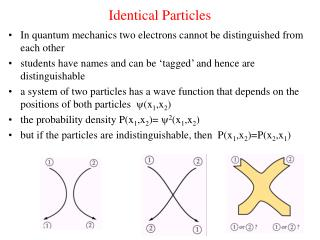Download PresentationIdentical Particles

Loading in 2 Seconds...

# Identical Particles - PowerPoint PPT Presentation

Identical Particles. In quantum mechanics two electrons cannot be distinguished from each other students have names and can be ‘tagged’ and hence are distinguishable a system of two particles has a wave function that depends on the positions of both particles (x 1 ,x 2 )I am the owner, or an agent authorized to act on behalf of the owner, of the copyrighted work described.
Download Presentation## Identical Particles

An Image/Link below is provided (as is) to download presentation

Download Policy: Content on the Website is provided to you AS IS for your information and personal use and may not be sold / licensed / shared on other websites without getting consent from its author.While downloading, if for some reason you are not able to download a presentation, the publisher may have deleted the file from their server.

- - - - - - - - - - - - - - - - - - - - - - - - - - E N D - - - - - - - - - - - - - - - - - - - - - - - - - -
Presentation Transcript
1. Identical Particles • In quantum mechanics two electrons cannot be distinguished from each other • students have names and can be ‘tagged’ and hence are distinguishable • a system of two particles has a wave function that depends on the positions of both particles (x1,x2) • the probability density P(x1,x2)= 2(x1,x2) • but if the particles are indistinguishable, then P(x1,x2)=P(x2,x1)

2. Identical Particles • P(x1,x2)=P(x2,x1) => (x1,x2)= (x2,x1) • if (x1,x2)=+ (x2,x1) => symmetric • if (x1,x2)= -(x2,x1) => anti-symmetric • if n(x1) is the wave function for particle 1 in state n and n(x2) is the wave function for particle 2 in state n • then the symmetric wave function is S(x1,x2)= A[n(x1) n(x2) + n(x2) n(x1) ] • the anti-symmetric wave function is A(x1,x2)= A[n(x1) n(x2) - n(x2) n(x1) ] • note: A(x1,x1)= 0 • this is the Pauli principle!!!

3. Pauli Principle • There are two types of particles in nature • fermions have an anti-symmetric wave function • bosons have a symmetric wave function • electrons are fermions => no two electrons can be in the same quantum state • this principle explains the periodic table, properties of metals, and the stability of stars • electrons repel but not because they are charged!

4. What is the ground-state energy of ten noninteracting fermions, such as neutrons, in a one-dimensional box of length L? (Because the quantum number associated with spin can have two values, each spatial state can hold two neutrons.) For fermions, such as neutrons for which the spin quantum number is 1/2, two particles can occupy the same spatial state. Consequently, the lowest total energy for the 10 fermions is E = 2E1(1 + 4 + 9 + 16 + 25) = 55h2 /4mL2 . En = n2h2/8mL2

5. Problems • I · A mass of 10-6 g is moving with a speed of about 10-1 cm/s in a box of length 1 cm. Treating this as a one-dimensional particle in a box, calculate the approximate value of the quantum number n. • 1. Write the energy of the particle E = (1/2)mv2 • 2. Write the expression for En = n2h2/8mL2 • 3. Solve for n = 2mvL/h= 3.02 x 1019 • II · (a) For the classical particle above, find x and  p, assuming that these uncertainties are given by  x/L = 0.01% and p/p = 0.01%. • (b) What is ( x p)/ħ ? • (a) x = 10-4x10-2 m = 10-6 m p = 10-4(mv) = 10-4x10-9x10-3 kg . m/s = 10-16 kg . m/s. • (b) x p/ ħ = 10-22 /1.05 x10-34 = 0.948 x1012 ».5

6. Expectation Values • We have that the probability of finding a particle near x is P(x)dx=2(x)dx • if we make a large number of measurements of position, then the average value of such measurements is the expectation value <x>

7. Expectation Values • What is <x> for a particle in its ground state in a box of length L? • What is <x2> for a particle in its ground state in a box of length L?

8. Problems • True or false: • (a) It is impossible in principle to know precisely the position of an electron. • False • (b) A particle that is confined to some region of space cannot have zero energy. • True • (c) All phenomena in nature are adequately described by classical wave theory. • False • (d) The expectation value of a quantity is the value that you expect to measure. • False; it is the most probable value of the measurement.